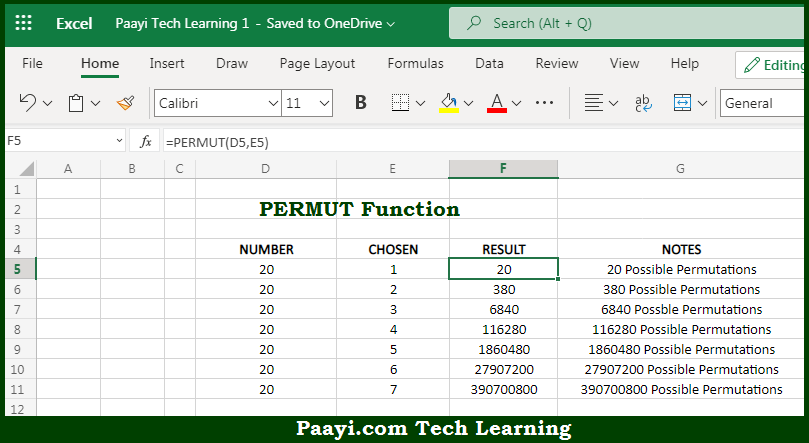# Learn How to Use Microsoft Excel PERMUT Function

Written by | 0 Comments | 699 Views

In this article, you will learn how to use the Microsoft Excel PERMUT function and its prime function in Microsoft Excel. You will also get to know the Microsoft Excel PERMUT function return value and syntax with the help of some examples.

Microsoft Excel PERMUT Function

The main purpose of the Microsoft Excel PERMUT function is to get the number of permutations without repetitions. That implies, with the help of PERMUT function you can able to return the number of permutations, which are the combinations where an order is significant for a given number of items. It should be noted that PERMUT function does not allow repetitions. In case you want to allow permutations with repetitions, use the PERMUTATIONA function. So, with the help of PERMUT function, you can able to get the number of permutations without repetitions.

Return Value of PERMUT Function

The return value will be the number of permutations as an integer.

Syntax of PERMUT Function

=PERMUT(number, number-chosen)

Where the arguments:

• number: This is the total number of items.
• number-chosen: This is the number of items in each combination.

## How to Use Microsoft Excel PERMUT Function?So we know that Microsoft Excel PERMUT function you can able to get the number of permutations without repetitions. That implies, with the help of PERMUT function you can able to return the number of permutations, which are the combinations where an order is significant for a given number of items. It should be noted that PERMUT function does not allow repetitions. In case you want to allow permutations with repetitions, use the PERMUTATIONA function. So, with the help of PERMUT function, you can able to get the number of permutations without repetitions.